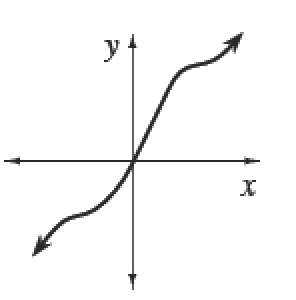### Home > APCALC > Chapter 3 > Lesson 3.3.4 > Problem3-135

3-135.

If a function $f$ is increasing, such as the one at right, what must be true about $f^\prime$

1. Explain your idea using the graph at right.

2. Use your idea to algebraically determine where $g(x) = 3x^2 − 3x + 1$ is increasing.If $f(x)$ is increasing, then slopes are positive so $f ^\prime(x) > 0$.

Use the Power Rule to find $f ^\prime(x)$.

Solve the inequality: $f ^\prime (x) > 0$.